# Chair of Software Engineering Einfhrung in die Programmierung

• Slides: 34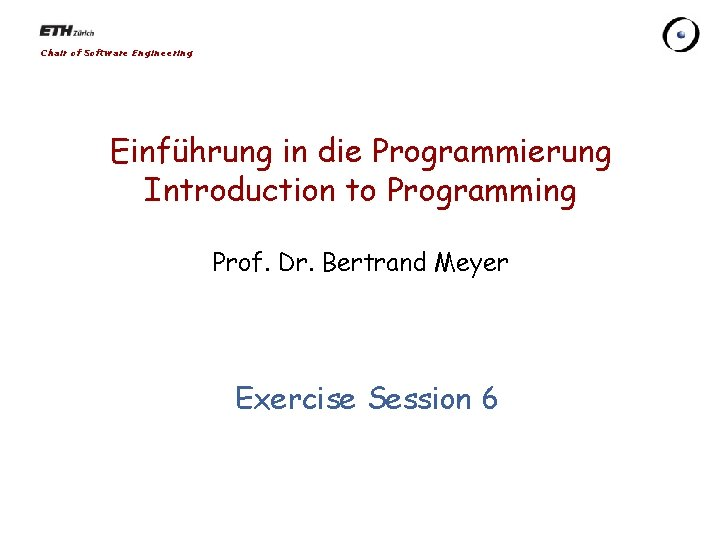Chair of Software Engineering Einführung in die Programmierung Introduction to Programming Prof. Dr. Bertrand Meyer Exercise Session 6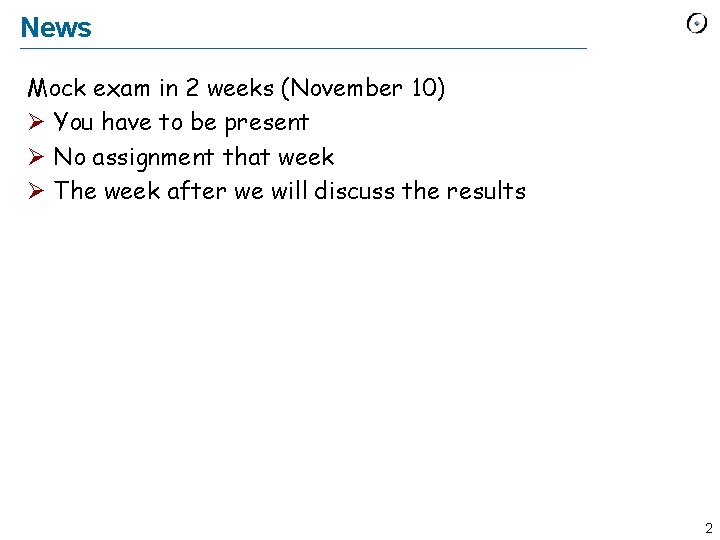News Mock exam in 2 weeks (November 10) Ø You have to be present Ø No assignment that week Ø The week after we will discuss the results 2Today Ø Conditional Ø Loop Ø Linked list 3Inside the routine body Ø The body of each routine consists of instructions (command calls, creations, assignments, etc. ) Ø In the programs you’ve seen so far they were always executed in the order they were written create passenger. make_with_route (Route 3, 1. 5) passenger. go passenger. set_reiterate (True) Paris. put_passenger (passenger) create tram. make_with_line (Line 1) tram. start Paris. put_tram (tram) Ø Programming languages have structures that allow you to change the order of execution 4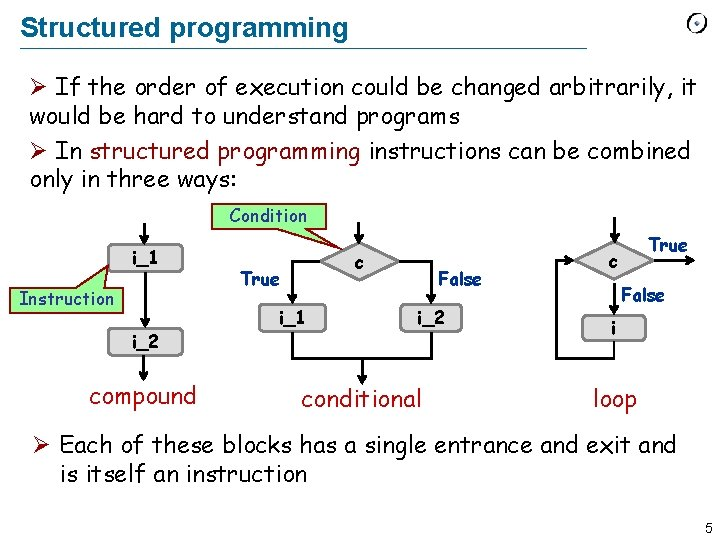Structured programming Ø If the order of execution could be changed arbitrarily, it would be hard to understand programs Ø In structured programming instructions can be combined only in three ways: Condition i_1 Instruction i_2 compound c True i_1 False i_2 conditional True c False i loop Ø Each of these blocks has a single entrance and exit and is itself an instruction 5Conditional Ø Basic syntax: Condition if c then Instruction i_1 else Instruction i_2 end Ø c is a boolean expression (e. g. , entity, query call of type BOOLEAN) Ø else-part is optional: if c then i_1 end 6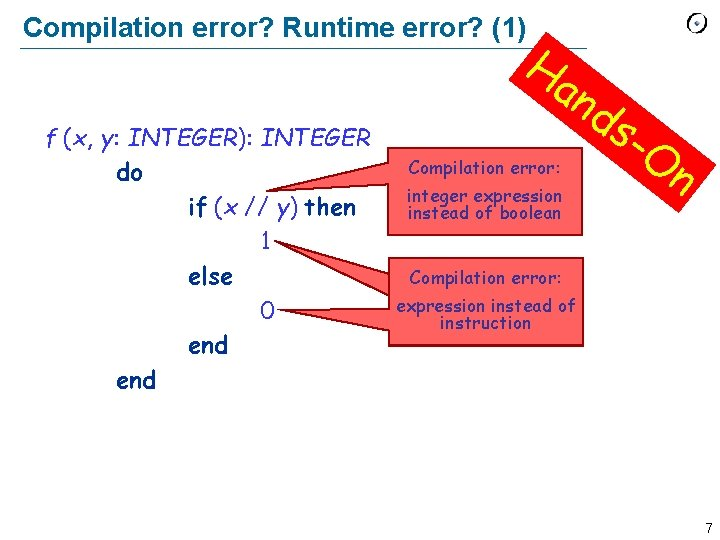Compilation error? Runtime error? (1) f (x, y: INTEGER): INTEGER do if (x // y) then 1 else 0 end Ha n ds Compilation error: integer expression instead of boolean -O n Compilation error: expression instead of instruction 7Compilation error? Runtime error? (2) f (x, y: INTEGER): INTEGER do if (False) then Result : = x // y end if (x /= 0) then Result : = y // x end Ha n ds -O n Everything is OK (during both compilation and runtime) 8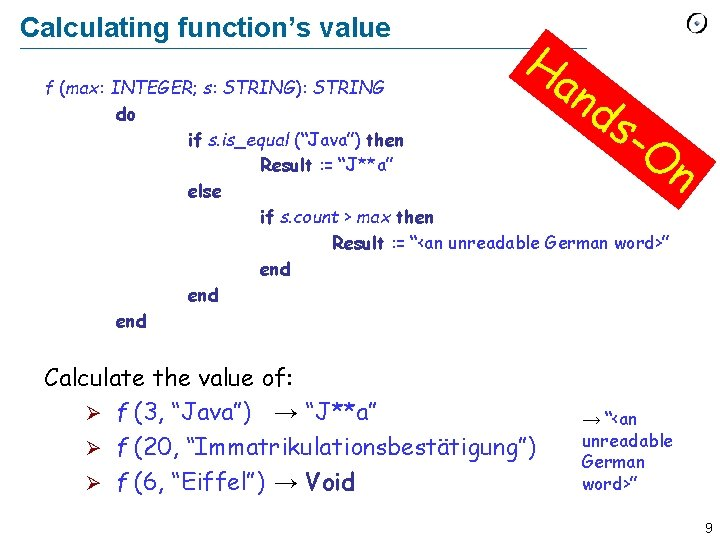Calculating function’s value Ha n ds f (max: INTEGER; s: STRING): STRING do if s. is_equal (“Java”) then Result : = “J**a” else if s. count > max then Result : = “<an unreadable German word>” end end Calculate the value of: Ø f (3, “Java”) → “J**a” Ø f (20, “Immatrikulationsbestätigung”) Ø f (6, “Eiffel”) → Void -O n → “<an unreadable German word>” 9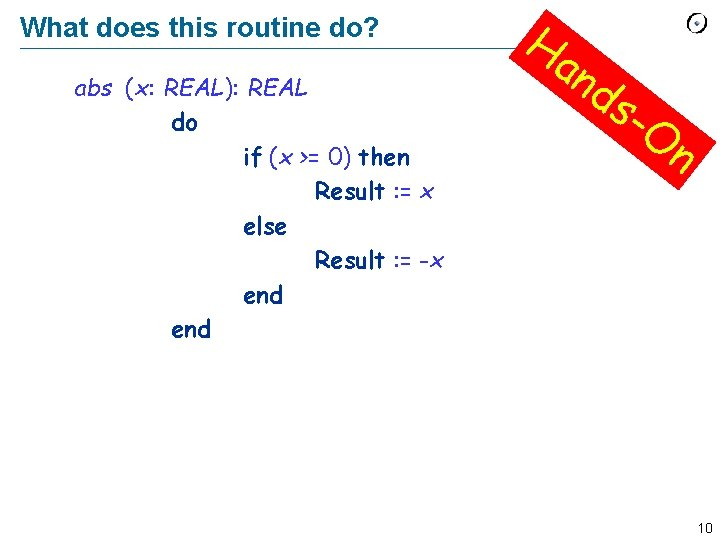What does this routine do? f (x: REAL): REAL abs do if (x >= 0) then Result : = x else Result : = -x end Ha n ds -O n 10Write a routine. . . Ha n ds Ø. . . that computes the maximum of two integers: max (a, b: INTEGER): INTEGER Ø. . . that increases time by one second inside class TIME: class TIME hour, minute, second: INTEGER -O n second_forth do. . . end 11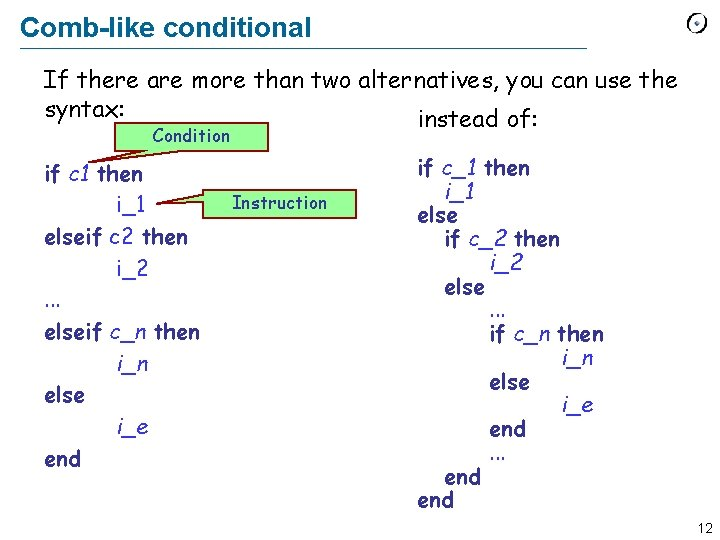Comb-like conditional If there are more than two alternatives, you can use the syntax: instead of: Condition if c 1 then i_1 elseif c 2 then i_2. . . elseif c_n then i_n else i_e end Instruction if c_1 then i_1 else if c_2 then i_2 else. . . if c_n then i_n else i_e end. . . end 12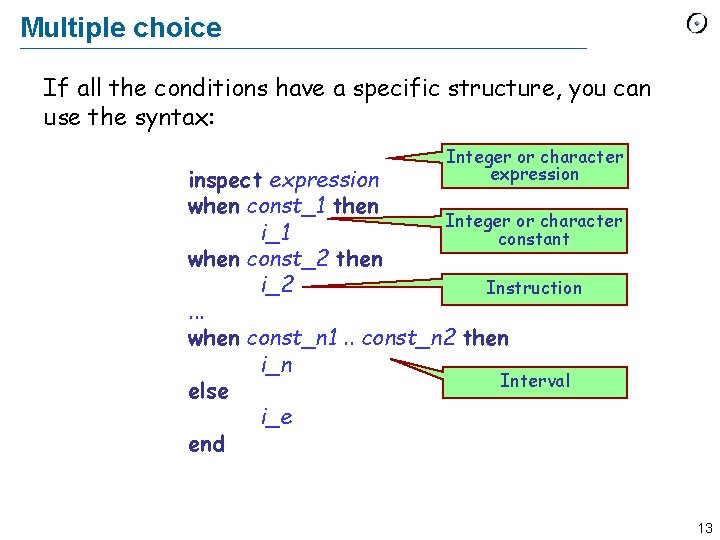Multiple choice If all the conditions have a specific structure, you can use the syntax: Integer or character expression inspect expression when const_1 then Integer or character i_1 constant when const_2 then i_2 Instruction. . . when const_n 1. . const_n 2 then i_n Interval else i_e end 13Lost in conditions Rewrite the following multiple choice: Ø using a comb-like conditional Ø using nested conditionals Ha n ds -O n inspect user_choice when 0 then print (“Here is your hamburger”) when 1 then print (“Here is your Coke”) else print (“Sorry, not on the menu today!”) end 14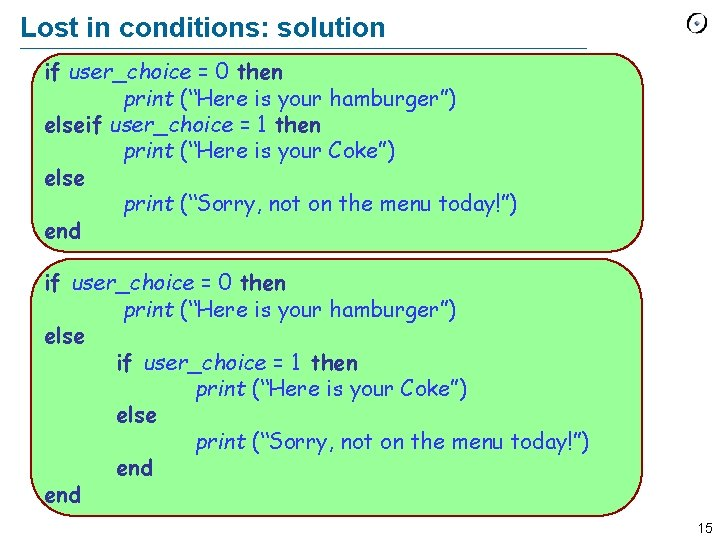Lost in conditions: solution if user_choice = 0 then print (“Here is your hamburger”) elseif user_choice = 1 then print (“Here is your Coke”) else print (“Sorry, not on the menu today!”) end if user_choice = 0 then print (“Here is your hamburger”) else if user_choice = 1 then print (“Here is your Coke”) else print (“Sorry, not on the menu today!”) end 15Loop Syntax: from initialization invariant inv until exit_condition loop body variant var end Instruction Optional Boolean expression Instruction Integer expression 16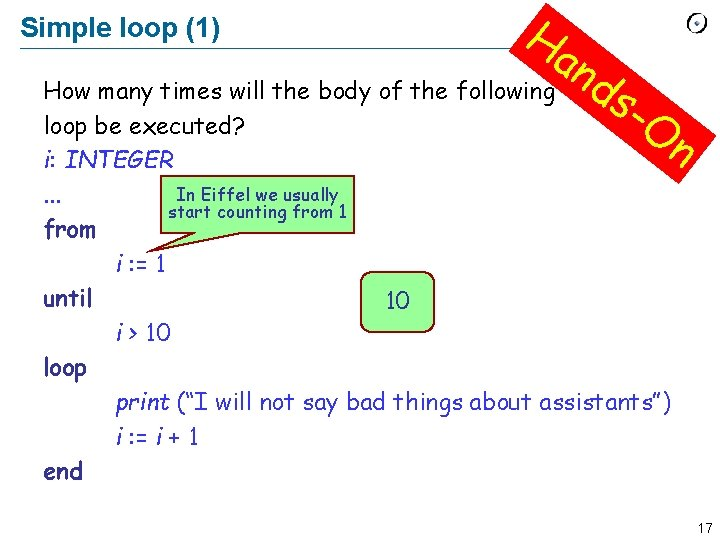Simple loop (1) Ha n ds How many times will the body of the following loop be executed? i: INTEGER In Eiffel we usually. . . start counting from 1 from i : = 1 until 10 i > 10 loop print (“I will not say bad things about assistants”) i : = i + 1 end -O n 17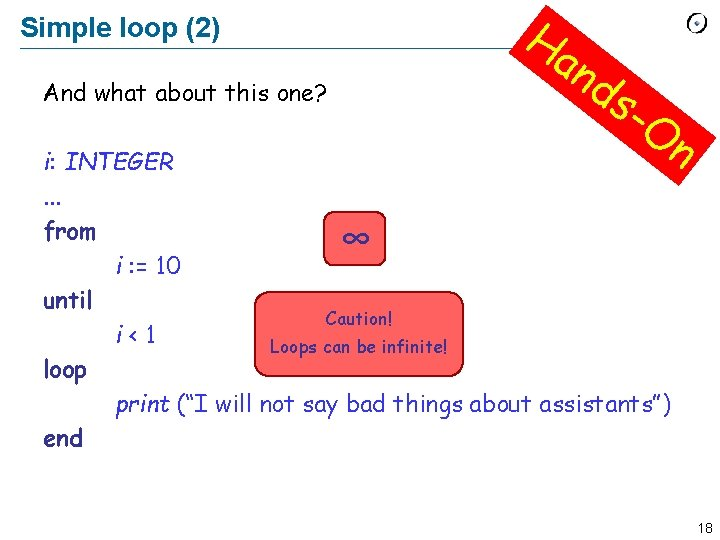Simple loop (2) And what about this one? Ha n ds -O n i: INTEGER. . . from ∞ i : = 10 until Caution! i<1 Loops can be infinite! loop print (“I will not say bad things about assistants”) end 18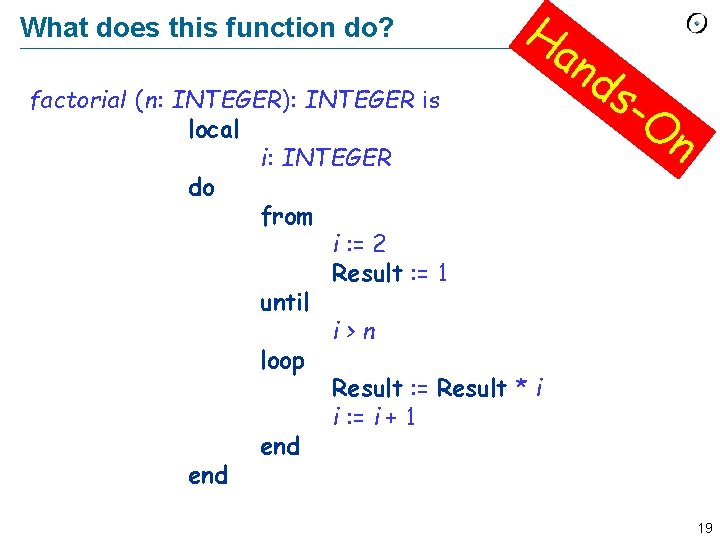What does this function do? Ha n factorialf (n: INTEGER): INTEGER is local i: INTEGER do from i : = 2 Result : = 1 until i>n loop Result : = Result * i i : = i + 1 end ds -O n 19Invariant and variant Loop invariant (do not mix with class invariant) Ø holds after execution of from clause and after each execution of loop clause Ø captures how the loop iteratively solves the problem: e. g. “to calculate the sum of all n elements in a list, on each iteration i (i = 1. . n) the sum of first i elements is obtained” Loop variant Ø integer expression that is nonnegative after execution of from clause and after each execution of loop clause and strictly decreases with each iteration Ø a loop with a variant can not be infinite (why? ) 20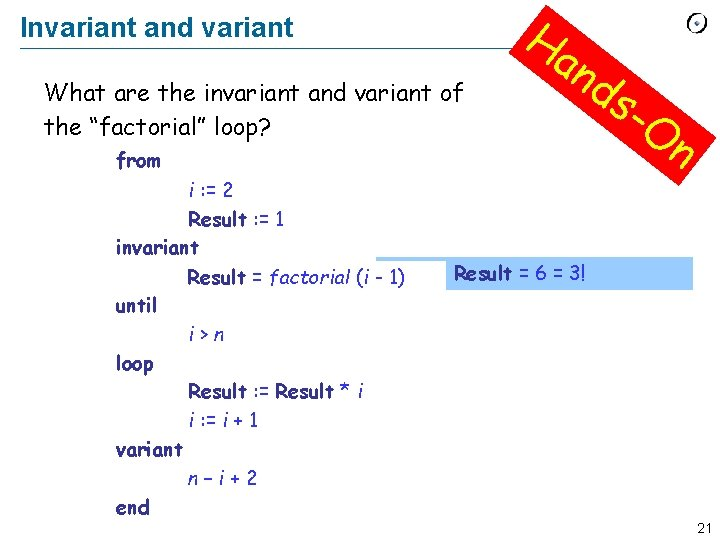Invariant and variant What are the invariant and variant of the “factorial” loop? Ha n from i : = 2 Result : = 1 invariant = 4; 2; 3; ? Result = factorial (i -i 1) until i>n loop Result : = Result * i i : = i + 1 variant n? – i + 2 end ds -O n Result = 6 1 == 1! 2 2! 3! 21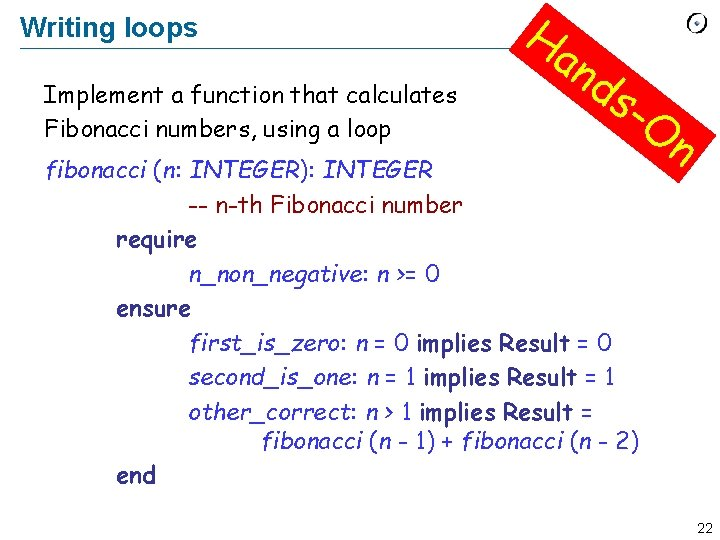Writing loops Implement a function that calculates Fibonacci numbers, using a loop Ha n ds -O fibonacci (n: INTEGER): INTEGER -- n-th Fibonacci number require n_non_negative: n >= 0 ensure first_is_zero: n = 0 implies Result = 0 second_is_one: n = 1 implies Result = 1 other_correct: n > 1 implies Result = fibonacci (n - 1) + fibonacci (n - 2) end n 22Writhing loops (solution) fibonacci (n: INTEGER): INTEGER local a, b, i: INTEGER do if n <= 1 then Result : = n else from a : = fibonacci (0) b : = fibonacci (1) i : = 1 invariant a = fibonacci (i - 1) b = fibonacci (i ) until i=n loop Result : = a + b a : = b b : = Result i : = i + 1 variant n-i end end Ha n ds -O n 23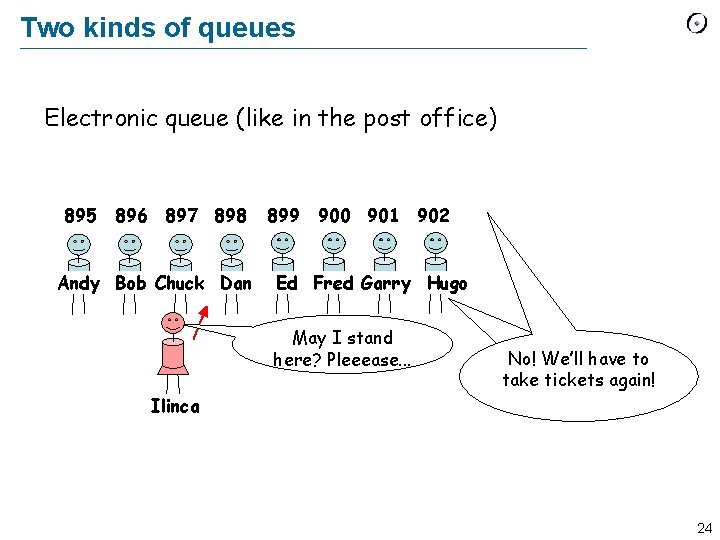Two kinds of queues Electronic queue (like in the post office) 895 896 897 898 Andy Bob Chuck Dan 899 900 901 902 Ed Fred Garry Hugo May I stand here? Pleeease. . . No! We’ll have to take tickets again! Ilinca 24Two kinds of queues Live queue Chuck is after me Bob is after me Dan is after me Andy Bob Chuck Dan . . . Ed Fred Garry Hugo I am the last May I stand here? Pleeease. . . Sure! Just remember that Dan is after you Ilinca 25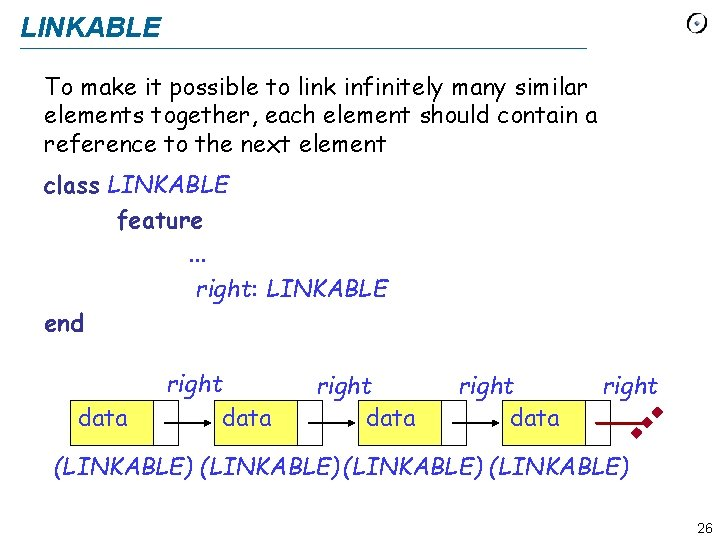LINKABLE To make it possible to link infinitely many similar elements together, each element should contain a reference to the next element class LINKABLE feature. . . right: LINKABLE end data right (LINKABLE) 26INT_LINKABLE class INT_LINKABLE create put feature item: INTEGER put (i: INTEGER) do item : = i end right: INT_LINKABLE put_right (other: INT_LINKABLE) do right : = other end 27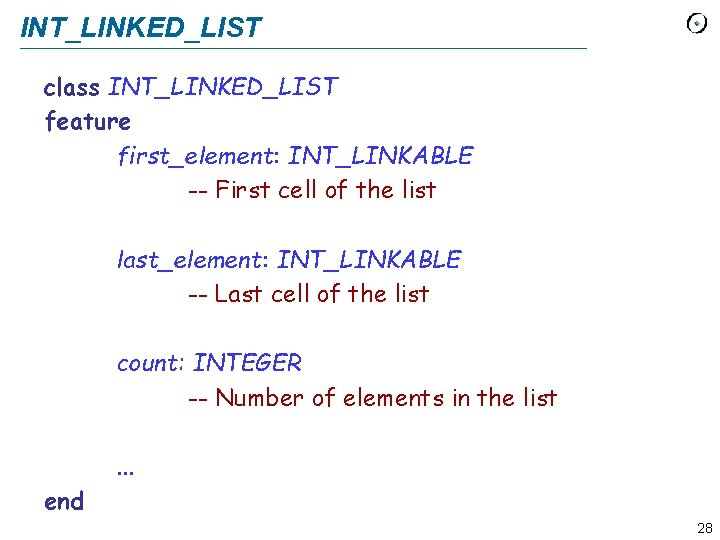INT_LINKED_LIST class INT_LINKED_LIST feature first_element: INT_LINKABLE -- First cell of the list last_element: INT_LINKABLE -- Last cell of the list count: INTEGER -- Number of elements in the list. . . end 28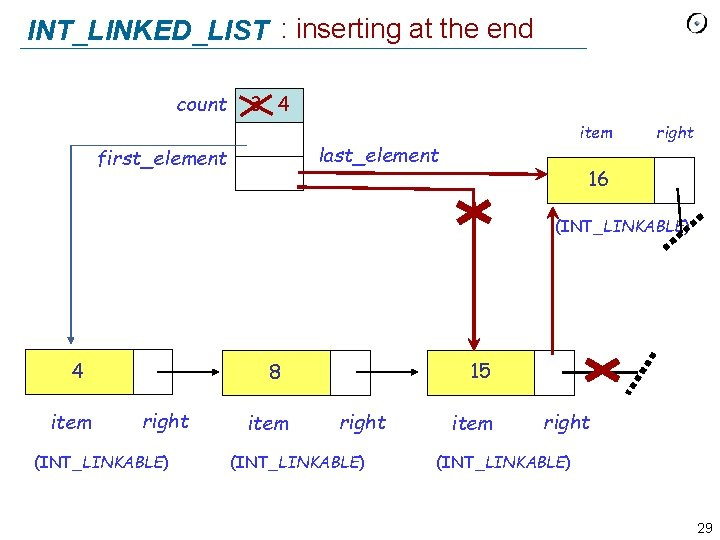INT_LINKED_LIST : inserting at the end count 3 4 item last_element first_element right 16 (INT_LINKABLE) 4 item 15 8 right (INT_LINKABLE) item right (INT_LINKABLE) 29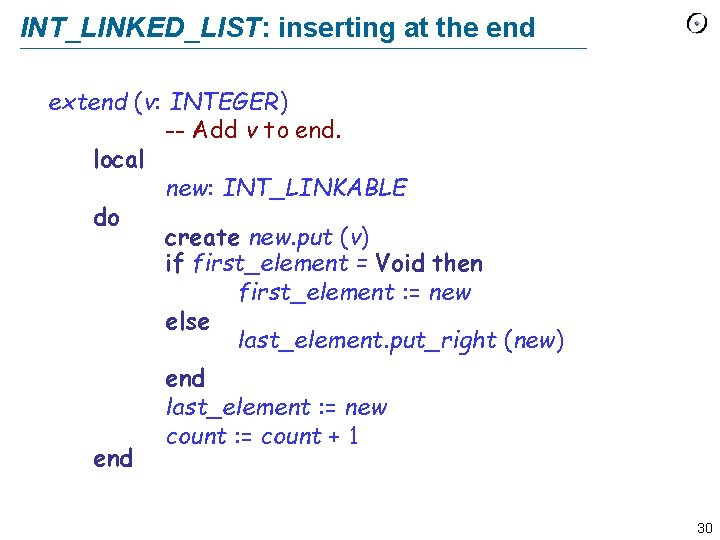INT_LINKED_LIST: inserting at the end extend (v: INTEGER) -- Add v to end. local new: INT_LINKABLE do create new. put (v) if first_element = Void then first_element : = new else last_element. put_right (new) end last_element : = new count : = count + 1 30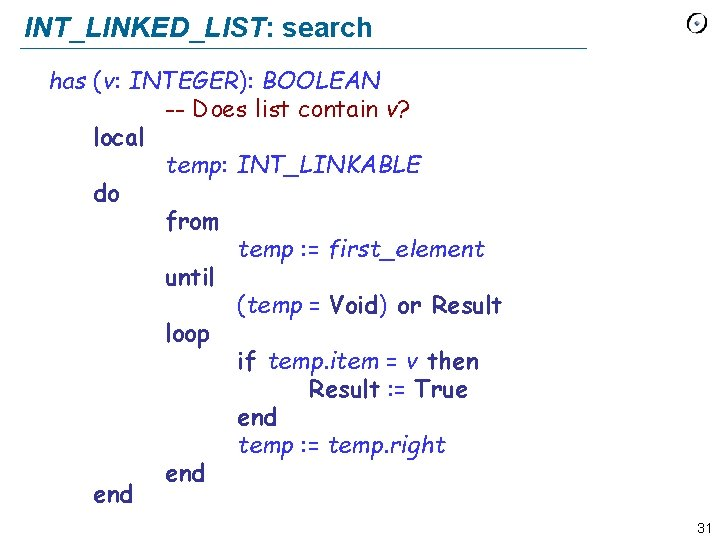INT_LINKED_LIST: search has (v: INTEGER): BOOLEAN -- Does list contain v? local temp: INT_LINKABLE do from temp : = first_element until (temp = Void) or Result loop if temp. item = v then Result : = True end temp : = temp. right end 31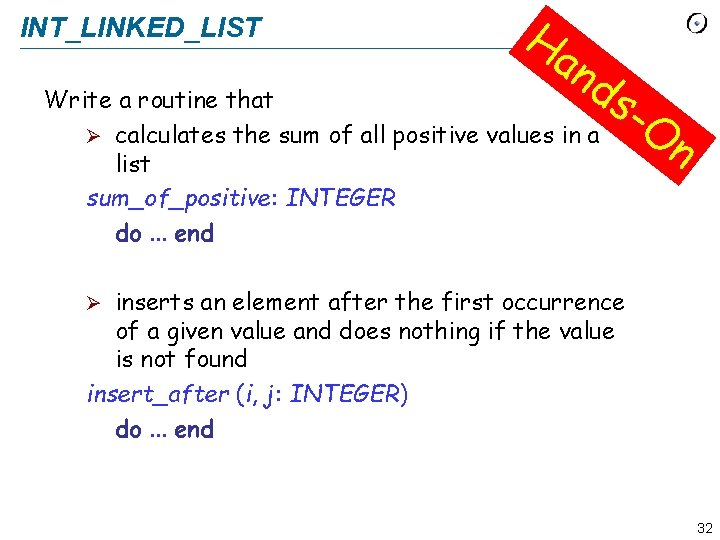INT_LINKED_LIST Ha n ds Write a routine that Ø calculates the sum of all positive values in a list sum_of_positive: INTEGER do. . . end -O n inserts an element after the first occurrence of a given value and does nothing if the value is not found insert_after (i, j: INTEGER) do. . . end Ø 32INT_LINKED_LIST: sum_of_positive: INTEGER -- Some of positive elements local temp: INT_LINKABLE do from temp : = first_element until temp = Void loop if temp. item > 0 then Result : = Result + temp. item end temp : = temp. right end 33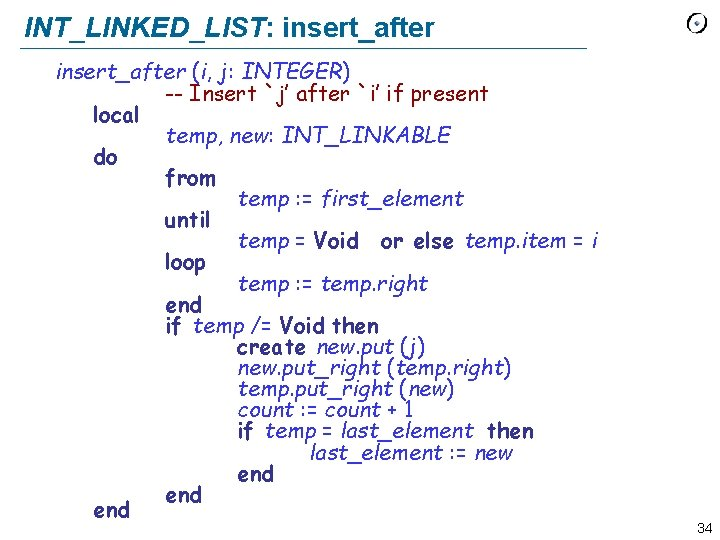INT_LINKED_LIST: insert_after (i, j: INTEGER) -- Insert `j’ after `i’ if present local temp, new: INT_LINKABLE do from temp : = first_element until temp = Void or else temp. item = i loop temp : = temp. right end if temp /= Void then create new. put (j) new. put_right (temp. right) temp. put_right (new) count : = count + 1 if temp = last_element then last_element : = new end end 34